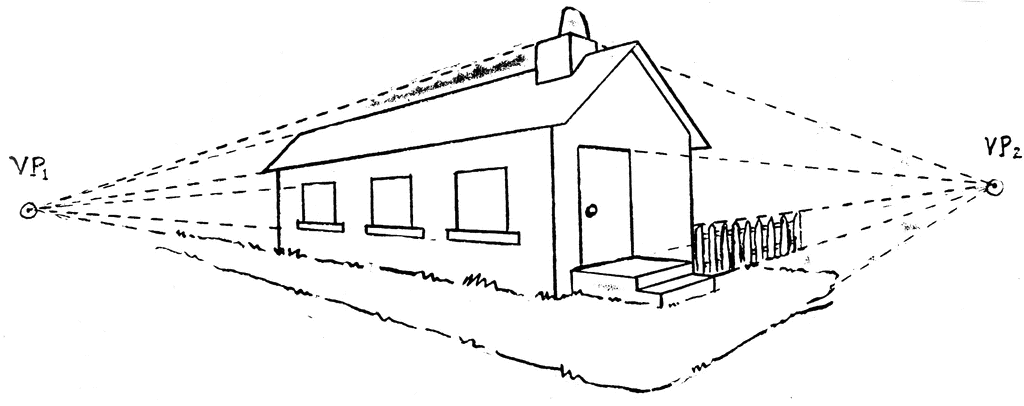$z\begin{bmatrix} p_x \\ p_y \\ 1 \end{bmatrix} = \mathbf{K}\cdot (\mathbf{o} + \lambda\mathbf{d}).$\begin{align} \mathbf{n}_{u} &= \boldsymbol{u}_{1}\times \boldsymbol{u}_{2}\\ \mathbf{n}_{v} &= \boldsymbol{v}_{1}\times \boldsymbol{v}_{2}. \end{align}

$\mathbf{d}=\mathbf{n}_{u}\times \mathbf{n}_{v}.$

$D\left(\mathbf{d}_{1},\mathbf{d}_{2}\right)=\left\langle \frac{\mathbf{d}_{1}}{\left\Vert \mathbf{d}_{1}\right\Vert },\frac{\mathbf{d}_{2}}{\left\Vert \mathbf{d}_{2}\right\Vert }\right\rangle$

$\mathbf{K}=\begin{bmatrix} f & 0 & o_{x}\\ & f & o_{y}\\ & & 1 \end{bmatrix}.$

\begin{align*} z_{u_i}\hat{u}_{i} & =\mathbf{K}u_{i}\\ z_{v_i}\hat{v}_{i} & =\mathbf{K}v_{i}, \end{align*}

\begin{align*} \mathbf{d} & =\mathbf{n}_{u}\times \mathbf{n}_{v}\\ & =\left(u_{1}\times u_{2}\right)\times\left(v_{1}\times v_{2}\right)\\ & \propto \left[\left(\mathbf{K}^{-1}\hat{u}_{1}\right)\times\left(\mathbf{K}^{-1}\hat{u}_{2}\right)\right]\times\left[\left(\mathbf{K}^{-1}\hat{v}_{1}\right)\times\left(\mathbf{K}^{-1}\hat{v}_{2}\right)\right]\\ & \stackrel{1}{=}\left[\mathbf{K}^{T}\left(\hat{u}_{1}\times\hat{u}_{2}\right)\right]\times\left[\mathbf{K}^{T}\left(\hat{v}_{1}\times\hat{v}_{2}\right)\right]\\ & \stackrel{2}{=}\mathbf{K}^{-1}\left[\left(\hat{u}_{1}\times\hat{u}_{2}\right)\times\left(\hat{v}_{1}\times\hat{v}_{2}\right)\right]\\ & =: \mathbf{K}^{-1}\left(\hat{\bf n}_{u}\times\hat{\bf n}_{v}\right)\\ & =: \mathbf{K}^{-1}\hat{\bf d}. \end{align*}

\begin{align*} \hat{\bf d}^T_{1}\mathbf{K}^{-T}\mathbf{K}^{-1}\hat{\bf d}_{2} & =0\\ \hat{\bf d}^T_{2}\mathbf{K}^{-T}\mathbf{K}^{-1}\hat{\bf d}_{3} & =0\\ \hat{\bf d}^T_{3}\mathbf{K}^{-T}\mathbf{K}^{-1}\hat{\bf d}_{1} & =0. \end{align*}

$\mathbf{S} := \mathbf{K}^{-T}\mathbf{K}^{-1}=\frac{1}{f^{2}}\begin{bmatrix}1 & 0 & -o_{x}\\ 0 & 1 & -o_{y}\\ -o_{x} & -o_{y} & o_{x}^{2}+o_{y}^{2}+f^{2} \end{bmatrix}$

$\min_{f,o_{x},o_{y}}\sum_{i,j}\ \left|\hat{\bf d}^{T}_{i}\mathbf{S}\hat{\bf d}_{j}\right|$

(原文地址: Yichao Zhou’s Blog)